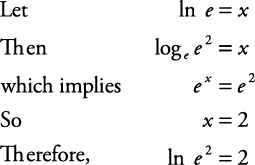## Logarithmic Functions

Logarithmic functions are the inverses of exponential functions, and any exponential function can be expressed in logarithmic form. Similarly, all logarithmic functions can be rewritten in exponential form. Logarithms are really useful in permitting us to work with very large numbers while manipulating numbers of a much more manageable size.

If x = 2 y were to be solved for y, so that it could be written in function form, a new word or symbol would need to be introduced. If x = 2 y , then y = (the power on base 2) to equal x. The word logarithm, abbreviated log, is introduced to satisfy this need.

y = (the power on base 2) to equal x

This equation is rewritten as y = log 2 x.

This is read as “ y equals the log of x, base 2” or “ y equals the log, base 2, of x.”

A logarithmic function is a function of the formwhich is read “ y equals the log of x, base b” or “ y equals the log, base b, of x.”In both forms, x > 0 and b > 0, b ≠ 1. There are no restrictions on y.

##### Example 1

Rewrite each exponential equation in its equivalent logarithmic form. The solutions follow.

1. 5 2 = 25

2.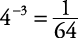3.1.2.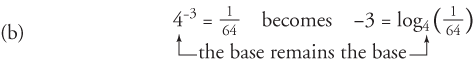3.##### Example 2

Rewrite each logarithmic equation in its equivalent exponential form. The solutions follow.

1. log 636 = 2

2. log am = p

1.2.##### Example 3

Solve the following equations, if possible.

1. log 749 = y

2.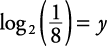3. log y 8 = 3

4. log 4 y = –2

5. log 3(–9) = y

1.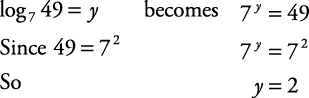2.3.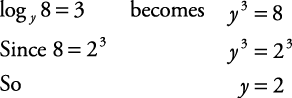4.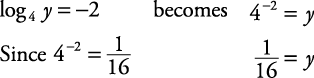5.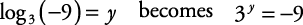This is not possible, since 3 y will always be a positive result. Recall that logarithms have only a positive domain; therefore, –9 is not in the domain of a logarithm.

The bases used most often when working with logarithms are base 10 and base e. (The letter e represents an irrational number that has many applications in mathematics and science. The value of e is approximately 2.718281828 …) Log base 10, log 10, is known as the common logarithm and is written as log, with the base not written but understood to be 10. Log base e, log e , is known as the natural logarithm and is written as ln.

##### Example 5

Find the following logarithms.

1. log 100

2. log 10,000

3. log 0.1

4. ln e

5. ln e 2

1.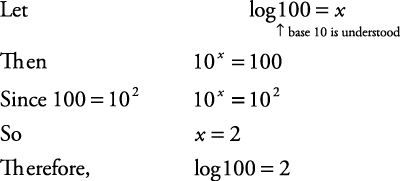2.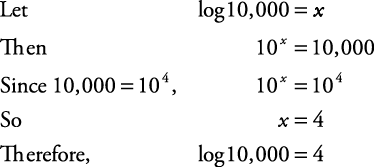3.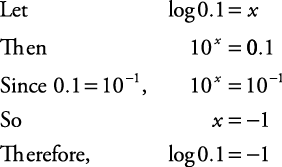4.5.